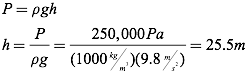# Pressure

Everyone’s been under pressure at one time or another, or in certain circumstances have really “felt the pressure.” From a scientific perspective, however, pressure has a very specific definition, and its exploration leads to some very important applications.

In physics, pressure is the effect of a force acting upon a surface. Mathematically, it is a scalar quantity calculated as the force applied per unit area, where the force applied is always perpendicular to the surface. The SI unit of pressure, a Pascal (Pa), is equivalent to a N/m2.All states of matter can exert pressure. When you walk across an ice-covered lake, you are applying a pressure to the ice equal to the force of gravity on your body (your weight) divided by the area over which you’re contacting the ice. This is why it is important to spread your weight out when traversing fragile surfaces. Your odds of breaking through the ice go up tremendously if you walk across the ice in high heels, as the small area contacting the ice leads to a high pressure. This is also the reason snow shoes have such a large area. They are designed to reduce the pressure applied to the top crust of snow so that you can walk more easily without sinking into snow drifts.Fluids, also, can exert pressure. All fluids exert outward pressure in all directions on the sides of any container holding the fluid. Even the Earth’s atmosphere exerts pressure, which you are experiencing right now. The pressures inside and outside your body are so well balanced, however, that you rarely notice the 101,325 Pascals due to the atmosphere (approximately 10N/cm2). If you ride in an airplane and change altitude (and therefore pressure) quickly, you may have experienced a “popping” sensation in your ears — this is due to the pressure inside your ear balancing the pressure outside your ear in a transfer of air through small tubes that connect your inner ear to your throat.

Question: Air pressure is approximately 100,000 Pascals. What force is exerted on this book when it is sitting flat on a desk? The area of the book’s cover is 0.035 m2.

Answer:Question: A fisherman with a mass of 75kg falls asleep on his four-legged chair of mass 5 kg. If each leg of the chair has a surface area of 2.5×10-4 m2 in contact with the ground, what is the average pressure exerted by the fisherman and chair on the ground?Answer: The force applied is the force of gravity, therefore we can write:Question: A scale which reads 0 in the vacuum of space is placed on the surface of planet Physica. On the planet’s surface, the scale indicates a force of 10,000 Newtons. Calculate the surface area of the scale, given that atmospheric pressure on the surface of Physica is 80,000 Pascals.

Answer:Question: Rank the following from highest pressure to lowest pressure upon the ground:

• The atmosphere at sea level
• A 7000-kg elephant with total area 0.5 m2 in contact with the ground
• A 65-kg lady in high heels with total area 0.005 m2 in contacting with the ground
• A 1600-kg car with a total tire contact area of 0.2 m2Answer: From highest pressure to lowest pressure:

• The elephant (137,000 Pa)
• The lady in high heels (127,000 Pa)
• The atmosphere (100,000 Pa)
• The car (78,400 Pa)

The pressure that a fluid exerts on an object submerged in that fluid can be calculated almost as simply. If the object is submersed to a depth (h), the pressure is found by multiplying the density of the fluid by the depth submerged, all multiplied by the acceleration due to gravity.This is known as the gauge pressure, because this is the reading you would observe on a pressure gauge. If there is also atmosphere above the fluid, such as the situation here on earth, you can determine the absolute pressure, or total pressure, by adding in the atmospheric pressure (P0), which is equal to approximately 100,000 Pascals.Question: Samantha spots buried treasure while scuba diving on her Caribbean vacation. If she must descend to a depth of 40 meters to examine the pressure, what gauge pressure will she read on her scuba equipment? The density of sea water is 1025 kg/m3.Answer:Question: What is the absolute pressure exerted on the diver in the previous problem by the water and atmosphere?Answer:Question: A diver’s pressure gauge reads 250,000 Pascals in fresh water (ρ=1000 kg/m3). How deep is the diver?

Answer: